# LOW CURRENT ELECTROLYSIS  OF WATER

Ph. M. Kanarev

E-mail: kanphil@mail.ru

An interest to hydrogen energetic is being increased of late years. It is explained by the fact that hydrogen is an inexhaustible and environmental-friendly energy carrier. But the implementation of these properties is slowed down by large energy consumption for its production from water.  The most modern Electrolyzers consume 4.0 kWh per cubic meter of this gas. Electrolysis process takes place by voltage of 1.6-2.0 V and current strength of dozens and hundreds of amperes. When one cubic meter of hydrogen is burnt, 3.55 kWh of energy is released .

Many laboratories in the world are busy solving a problem of a reduction of energy consumption for hydrogen production from water, but there are no significant results. In the meantime, a money-saving process of decomposition of water molecules into hydrogen and oxygen exists in the nature. This process takes place during photosynthesis. Hydrogen atoms are separated from water molecules and are used as connecting links while forming organic molecules, and oxygen is released into the air.

A question emerges: is it possible to model an electrolytical process of water decomposition into hydrogen and oxygen, which takes place during photosynthesis? A search of a reply to this question has resulted in a simple structure of a cell (Fig. 1), in which the process takes place by voltage of 1.5-2.0 V between the anode and the cathode and amperage of 0.02 amperes , .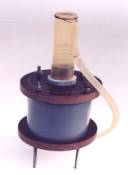Fig. 1. Model of a low current cell of the electrolyzer (at the stage of patenting)

The electrodes of the cell are made of steel. It helps to avoid the phenomena, which are appropriate to a galvanic cell. Nevertheless, at the cell electrodes a potential difference of nearly 0.1 V takes place in complete default of electrolytic solution in it. When the solution is charged, the potential difference is increased. The positive sign of the charge appears on the upper electrode always, and the negative sign appears on the lower one. If a direct current source generates pulses, gas output is increased.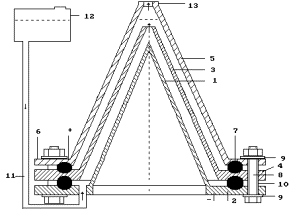Fig. 1a. Low current electrolyzer  (Patent # 2227817)

As a laboratory model of the low current electrolyzer cell generates small quantity of gases, a solution mass change definition method during the experiment and further calculation of released hydrogen and oxygen is the most reliable method of definition of their quantity.

It is known that a gram atom is equal to atomic mass of substance; a gram molecule is equal to molecular mass of substance. For example, the gram molecule of hydrogen in the water molecule is equal to two grams; the gram-atom of the oxygen atom is 16 grams. The gram molecule of water is equal to 18 grams. Hydrogen mass in a water molecule is 2x100/18=11.11%; oxygen mass is 16x100/18=88.89%; this ratio of hydrogen and oxygen is in one liter of water. It means that 111.11 grams of hydrogen and 888.89 grams of oxygen are in 1000 grams of water.

One liter of hydrogen weighs 0.09 g; one liter of oxygen weighs 1.47 g. It means that it is possible to produce 111.11/0.09=1234.44 liters of hydrogen and 888.89/1.47=604.69 liters of oxygen from one liter of water. It appears from this that one gram of water contains 1.23 liters of hydrogen. Energy consumption for production of 1000 liters of hydrogen is 4 kWh and for one liter 4 Wh. As it is possible to produce 1.234 liters of hydrogen from one gram of water, 1.234x4=4.94 Wh is spent for hydrogen production from one gram of water now.

Instruments and equipment used during the experiment

Special experimental low current electrolyzer (Fig. 3); voltmeter of the highest accuracy class (accuracy class of 0.2 GOST 9711-78); ammeter of the highest accuracy class (accuracy class of 0.2 GOST 9711-78)’ electronic scale with scale division value of 0.1 and 0.01 g; stop watch with scale division value of 0.1 s.

Table 1

Experimental results

Oscillogram samples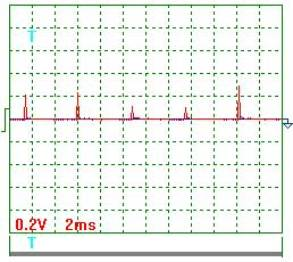Fig. 2. VoltageFig. 3. Voltage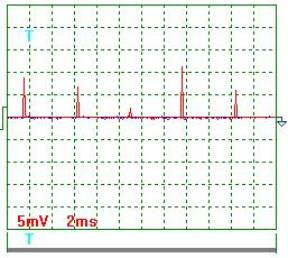Fig. 4. Current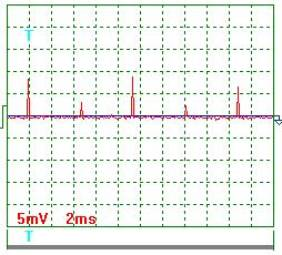Fig. 5. Current

Voltage oscillogram processing results (Figs 2 and 3). Taking into consideration the scale factor, which is equal to 10, we'll find a mean value of voltage pulse amplitude=[(0.20+0.24+0.12+0.10+0.30+0.18+0.16+0.12+0.30+ 0.24+0.30)/11] x10=2,05 V .

Pulse period Т=(24х2)/10=4.8 ms.

Pulse duration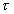=(2х1.45)/10=0.29 ms.

Pulse frequency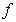=(1/0.001x4.8)=208.3 Hz.

Pulse period-to-pulse duration ratio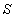=48/0.29=16.55.

Duty factor=0.5/16.55=0.0302.

Equivalent mean component of voltage pulses calculated according to the oscillograph readings=2.05х0.0302=0.062 V. At that time, the voltmeter readings were 11.0 V.

Current oscillogram processing results (Figs 4 and 5).  Taking into consideration the scale factor, which is equal to 10, and resistance of 0.1 Ohm resistor we'll find a mean value of current pulse amplitude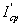={[(9.0+7.0+2.0+11.5 +6.0+8.5+3.5+9.0+2.5+6.5)/10]x10}/0.1=655мА =0.655 А.

Mean current in the electrolyzer supply circuit is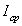=0.655х0.0302=0.01978А =0.02А. The ammeter readings are 0.02 А.

A question emerges at once: why is current value according to the readings of the ammeter and oscillograph the same and voltage value according to  the oscillograph readings is 177.4fold less than according to the voltmeter readings? A series of additional experiments accompanying this question is shown that a low current electrolyzer cell is a capacitor being discharged gradually under the influence of electrolytical processes, which take place in it. A value of this discharge is compensated by the pulses of voltage, which mean value is considerably less than a constant value of charge voltage of this capacitor.

Thus, the voltmeter shows a capacitor charge voltage value, and the  oscillograph shows a value of its recharge, which characterizes the energy consumed by the cell from the line. It appears from this that in order to calculate energy consumed by the low current electrolyzer cell from the line it is necessary to use voltage, which is registered not by the voltmeter, but by the oscillograph. As a result, energy consumption for hydrogen production from water in case of low current electrolysis are reduced not 12fold, but almost 2000fold.

Thus, a small value of current 0.02 A and voltage 0.062 V allows us to suppose that in the low current electrolyzer the water electrolysis process is similar to the process, which takes place during photosynthesis. At photosynthesis, hydrogen separated from the water molecule is used as a connecting link while organic molecule formation, and oxygen is released in the air. At low current electrolysis, both hydrogen and oxygen are released in the air.

Fruitfulness of this attractive hypothesis should be checked not once, but now it is the only one, which gives a satisfactory explanation of an unusual experimental result.

Note: gas release is clearly seen during several hours after the cell is disconnected from the line.

Conclusion

Energy efficiency index of the low current electrolysis should be refined, but in any case it will be greater than 10, that’s why there is every reason to think that a way to production of inexpensive hydrogen from water and transition to hydrogen energetic is opened.

REFERENCES

1. Kanarev Ph.M. The Foundation of  Physchemistry of  Microworld. The third edition. – Krasnodar: KSAU, 2003.  http://Kanarev.innoplaza.net (In Russian, Part 1, Part 2).

2. Kanarev Ph.M. The Foundation of  Physchemistry of  Microworld. The second edition. (In English). http://book.physchemistry.innoplaza.net

Webmaster: j_hartikka@hotmail.com

Low Current Electrolysis of Water by Prof. Kanarev

<< Kanarev´s Page